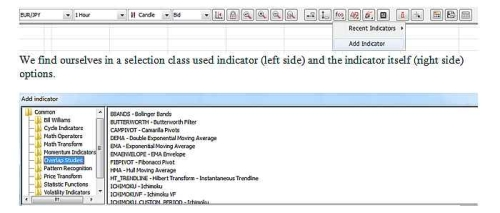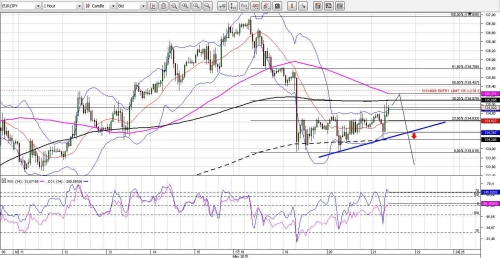The urgency of the problem:

Watching the trading strategies of novice traders often face the problem of misuse of the indicators and the definition of the input signals in the position of using them. The problem is not in the signals (they are mostly correct), and that the misused indicator does not function as an additional filter signal, and works as an indicator of duplicating the first signal. And this is not an additional filter signal and additional error duplication first signal.

The terms and definitions:

Referring to http://en.wikipedia.org/wiki/Multicollinearity we find classical concept associated with the analysis of statistical data and econometric models building.
Multicollinearity - mathematical statistics is a term used to describe a close correlation relationship between sampled for analysis of factors combined effect on the overall result.
In mathematics, the terms of collinearity - the relationship between the two studied Objects and multicollinearity relationship between more than two (many) of the test object. Last term was introduced by Nobel laureate Ragnar Frisch.

Introduction:

Multicollinearity is a problem also in technical analysis. It arises when we are not aware of the information using the same type more than once. This happens when the techniques used overlap. This is especially true beginners. They often do not question the work (as arranged as collects and reproduces data) technical indicators and thus make the mistake multicollinearity (in other words the signal duplication).
As noted John Bollinger: "The main rule for the successful use of technical analysis required to avoid multicollinearity between indicators. Multicollinearity - it's just a re-registration of the same information."

The main part:

Many trading platforms for removing the issue multekollinearnosti used partitioning reception indicators are not kolleniarnye classes. So add the specified function indicatorThat is, we must understand that the indicators used by different classes we remove the problem multicollinearity. If we use indicators of the same class, we simply duplicate the signal. And if the signal is wrong on one indicator, the second indicator (the same class) simply duplicate existing error, and will not serve us additional filter.
For example, we choose the class Momentum Indicators Two indicators RSI (14) and CCI (14).
Pay attention to the picture.Really one of the indicators of their signals would overlap one another. And when they are used at the same time we do not get an additional filter to the input position, and we get a duplicate version of the input signal.

Conclusion:

The most simple and at the same time the surest way to quickly identify collinearity between indicators - is to build their schedule. If substantially match the ups and downs one zone, the difference is negligible, they are collinear. In those cases, then there collinearity indicators, use only one of them.
What do you do when the lights differently display their information. Some of schedule, while others histogram or columns with different color. Then you need to calculate the correlation between the result sets of data, or simply analyze the formula for calculating the indicators. Having collinearity in one of the proposed methods we do not use overlapping signals.
The correct conclusion is always the same - you should not seek the confirmation signal between collinear indicators because it is not a confirmation signal and the error, which later can cost you cash costs.

Thank you for your interest in my article. Always your friend, Oleg - @salamandra
Translate to English Show original